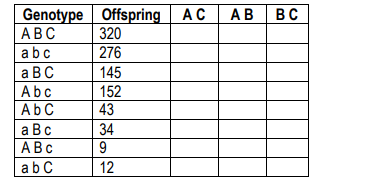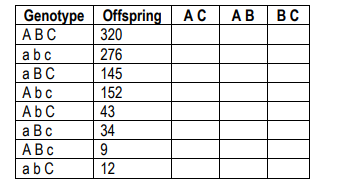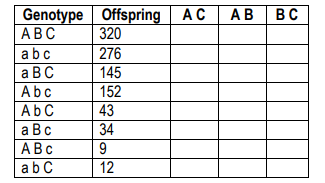Clutch Prep is now a part of Pearson
Ch.4 Genetic Mapping and LinkageWorksheetSee all chapters

# Trihybrid Cross

See all sections
Sections
Mapping Overview
Crossing Over and Recombinants
Mapping Genes
Trihybrid Cross
Multiple Cross Overs and Interference
Mapping with Markers
Positional Cloning

Concept #1: Trihybrid Cross

Practice: The following table shows data from a cross (ABC x abc) examining three genes (a, b, and c). Calculate the recombination frequency for A and BPractice: The following table shows data from a cross examining three genes (a, b, and c). Calculate the recombination frequency for B and CPractice: The following table shows data from a cross examining three genes (a, b, and c). Determine the order of genes